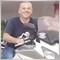• Übersicht
• Bewertungen (2)
• Diskussion (6)

# Urban

The Urban Expert Advisor automatically searches for the entry points based on the standard Parabolic SAR and CCI indicators. In case of unsuccessful entry, the EA maintains deals using the Martingale strategy. Special attention in this EA has been paid to the logic of placing orders, therefore it is able to withstand prolonged trends.

Unlike the Miami EA, in the Urban EA the TakeProfit is added to the average price (see the scheme on the last screenshot)

The default EA settings are for GBPUSD M5 with 5-digit quotes for the period from 01.01.15 to 01.01.16

In order to use the EA on 4-digit quotes, divide the values of the TakeProfit, PipsStep, MaxPipsStep parameters by 10 (expert advisor: for 5-digit quotes - TakeProfit=500, for 4 digit quotes - TakeProfit=50), but the results will differ at the same parameters.

Optimization cab be performed by Control points, example of the main parameters to optimize is provided in the screenshots

### Parameters

• UseRevers=true: Close profitable trade in case of a reverse signal
• UseBlock=true: If there is an opposite trend, the additional orders will not be opened until the trend weakens
• UseEndOfDay=true: If there is an open order left after 23:00, it will be closed regardless of the profit/loss level
• LotExponent: Coefficient for exponential multiplication of lots in the series for transferring to breakeven (optimize from 1.0 to 2.0 with any step)
• Lots: Open the initial deals with the same lots, if set to 0, the lot size if calculated based on Risk
• Risk: Determine the lot of the first order depending on the size of free margin. If Risk=1, the lot will be calculated as 0.01 lot per \$1000 of free margin for 5-digit quotes and 0.1 lot per \$1000 for 4-digit quotes
• TakeProfit: Take profit level from the opening price in points
• PipsStep: Distance of loss in points for opening the next order. Decreasing the PipStep increases the profitability and risk
• UseMaxPipsStep=true: Limit the maximum step when using the dynamic lot
• MaxPipsStep: Maximum allowed distance in points
• UseDynamicStep=true: Use dynamic step for opening the next order
• DynamicStep: Dynamic increase ratio of step between orders (optimize from 1 to 2 with any step).
• UseInd1=true: Use data of the Parabolic SAR to evaluate the trend
• RUseInd1=true: Use data of the Parabolic SAR to search for the reverse signal if UseRevers=true
• SARStep: Acceleration coefficient of the Parabolic SAR (Optimal parameters are close to standard)
• SARMaxStep: Maximum step of the Parabolic SAR (Optimal parameters are close to standard)
• UseInd2=true: Use data of the CCI to evaluate the trend
• RUseInd2=true: Use data of the CCI to search for the reverse signal if UseRevers=true
• CCIPeriod: Period of the CCI indicator
• CCIPrice: Applied price of the CCI (varies from 0 to 4 with step of 1)
• CCIMAPeriod: Period of the Moving Average, based on the CCI indicator
• CCIMAShift: Shift of the Moving Average, based on the CCI indicator
• CCIMAMethod: Averaging method of the Moving Average, based on the CCI indicator (varies from 0 to 3 with step of 1)
• TFrame: Timeframe of the indicators (0=current, 1=M5, 2=M15, 3=M30, 4=H1, 5=H4, 6=D1). It is convenient to use for the evaluation of the EA on different timeframes. Important! Optimization period in the tester must be smaller than the periods tested in the TFrame and ReversTFrame parameters
• ReversTFrame: Timeframe of the indicators to search for the reverse signal
• UseTime=true: Limit operation time of the EA (uses server time)
• HourStart: EA operation start time
• HourEnd: EA operation end time
• Slippage: Allowed price slippage in points
• MaxTrades: Maximum number of opened orders
• MagicNumber: Individual number of the EA

Note! The EA should be disabled before the events that may bring high volatility into the market (such events are always widely covered by the media), as strong rise/fall in the instrument may result in loss of deposit.

It is recommended to reoptimize the EA every six months59
2017.10.12 17:09

Up 20% for 3 days of using this EA. Works efficiently with my personal configuration. Minimal supervision. Best EA I tried so far. Excellent work Andray!566
2017.03.03 23:38

2017.03.03

10% of the profit from 22 February 2017. Max DD 0.28%. - RoboForex - cent account - VPS of about 20 ms.

I will update.